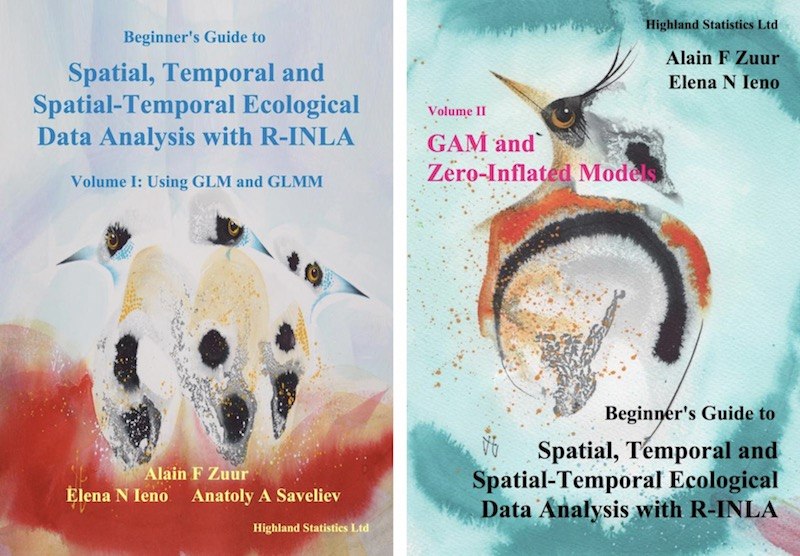#### Beginner's Guide to Spatial, Temporal and Spatial-Temporal Ecological Data Analysis with R-INLA (2017). Zuur, Ieno, Saveliev

This book consists of two volumes.

In Volume I we explain how to apply linear regression models, generalised linear models (GLM), and generalised linear mixed-effects models(GLMM) to spatial, temporal, and spatial-temporal data. The models that will be employed use the Gaussian and gamma distributions for continuous data, the Poisson and negative binomial distributions for count data, the Bernoulli distribution for absence–presence data, and the binomial distribution for proportional data.

In Volume II we apply zero-inflated models and generalised additive (mixed-effects) models to spatial and spatial-temporal data. Volume II is entitled  GAM and Zero-Inflated Models.

Outline of Volume I

In Chapter 2 we discuss an important topic: dependency. Ignoring this means that we have pseudoreplication. We present a series of examples and discuss how dependency can manifest itself.

We briefly discuss frequentist tools that are available for the analysis of temporal and spatial data in Chapters 3 and 4, and we will conclude that their application is rather limited, especially if non-Gaussian distributions are required. We will therefore consider alternative models, but these require Bayesian techniques.

In Chapter 5 we discuss linear mixed-effects models to analyse hierarchical (i.e. clustered or nested) data, and in Chapter 6 we outline how we add spatial and spatial-temporal dependency to regression models via spatial (and/or temporal) correlated random effects.

In Chapter 7 we introduce Bayesian analysis, Markov chain Monte Carlo techniques (MCMC), and Integrated Nested Laplace Approximation (INLA). INLA allows us to apply models to spatial, temporal, or spatial-temporal data.

In Chapters 8 through 16 we present a series of INLA examples. We start by applying linear regression and mixed-effects models in INLA (Chapters 8 and 9), followed by GLM examples in Chapter 10. In Chapters 11 through 13 we show how to apply GLM models on spatial data. In Chapter 14 we discuss time-series techniques and how to implement them in INLA. Finally, in Chapters 15 and 16 we analyse spatial-temporal models in INLA.

Outline of Volume II

In Chapter 18 we will explain how to deal with zero-inflated data. We introduce so-called zero-inflated Poisson (ZIP) models, zero-inflated negative binomial (ZINB) models, zero-altered Poisson (ZAP) models and zero-altered negative binomial (ZINB) models.

In Chapter 19 we extend the ZIP, ZINB, ZAP and ZANB models with spatial correlation. Both these chapters use a skate data set from South America. In the appendix accompanying Chapter 19 we also explain how to manipulate maps and create spatial polygons (e.g. for coastlines).

In Chapter 20 we revisit a data set with which we have been battling since 2006. It is about begging behaviour of owl nestlings. In Zuur (2009a) we applied linear mixed-effects models on it, and in Zuur et al. (2012a) we analysed it with a zero-inflated GLMM. Thanks to R-INLA we finally cracked this data set and apply a zero-inflated GAMM.

In Chapter 21 we analyse sandeel count data. This work came out of a consultancy project that we carried out for Wageningen Marine Research (The Netherlands) in 2017. Although the setup of the experiment is simple (approximately 400 sites sampled once per year, for 4 years), analysing these data and writing this chapter took about 30 days. This should give you an idea about the complexity of the statistical tools (zero-inflated GAMMs + spatial-temporal correlation) that we discuss in this book.

Chapter 22 is about zero-inflated bird densities sampled in the Labrador Sea, located between the Labrador Peninsula (Eastern Canada) and Greenland. This chapter is about the analysis of zero-inflated continuous data with spatial correlation. A zero-altered gamma model with spatial correlation is used.

In Chapter 23 we analyse coral reef data sampled around an island. A lot of misery comes together in this chapter: smoothers, zero-inflation and spatial dependency that should not cross land as benthic species that live in a coral reef do not walk over land! We will discuss barrier models (Bakka et al. 2018) which ensure that spatial correlation seeps around a barrier (in this case an island).

Up to Chapter 23 all data sets analysed were geostatistical data and not areal or lattice data. The reason for this is that most ecological data is geostatistical. In Chapter 24 we analyse aggregated tornado data in 102 counties in Illinois. This is areal data. We will use various CAR models (e.g. iCAR, BYM, BYM2) for zero-inflated spatial and spatial-temporal correlated data.

Data and R code VOLUME I

All data is freely available. All the R code is provided as well, except that a password is needed to open the zip files. The password is given in the Preface of Volume I (see page vi). In some chapters we are sourcing our support file HighstatLibV10.R.

Data and R code VOLUME II

All data is freely available. All the R code is provided as well, except that a password is needed to open the zip files. The password is given in the Preface of Volume II. In some chapters we are sourcing our support file HighstatLibV11.R.